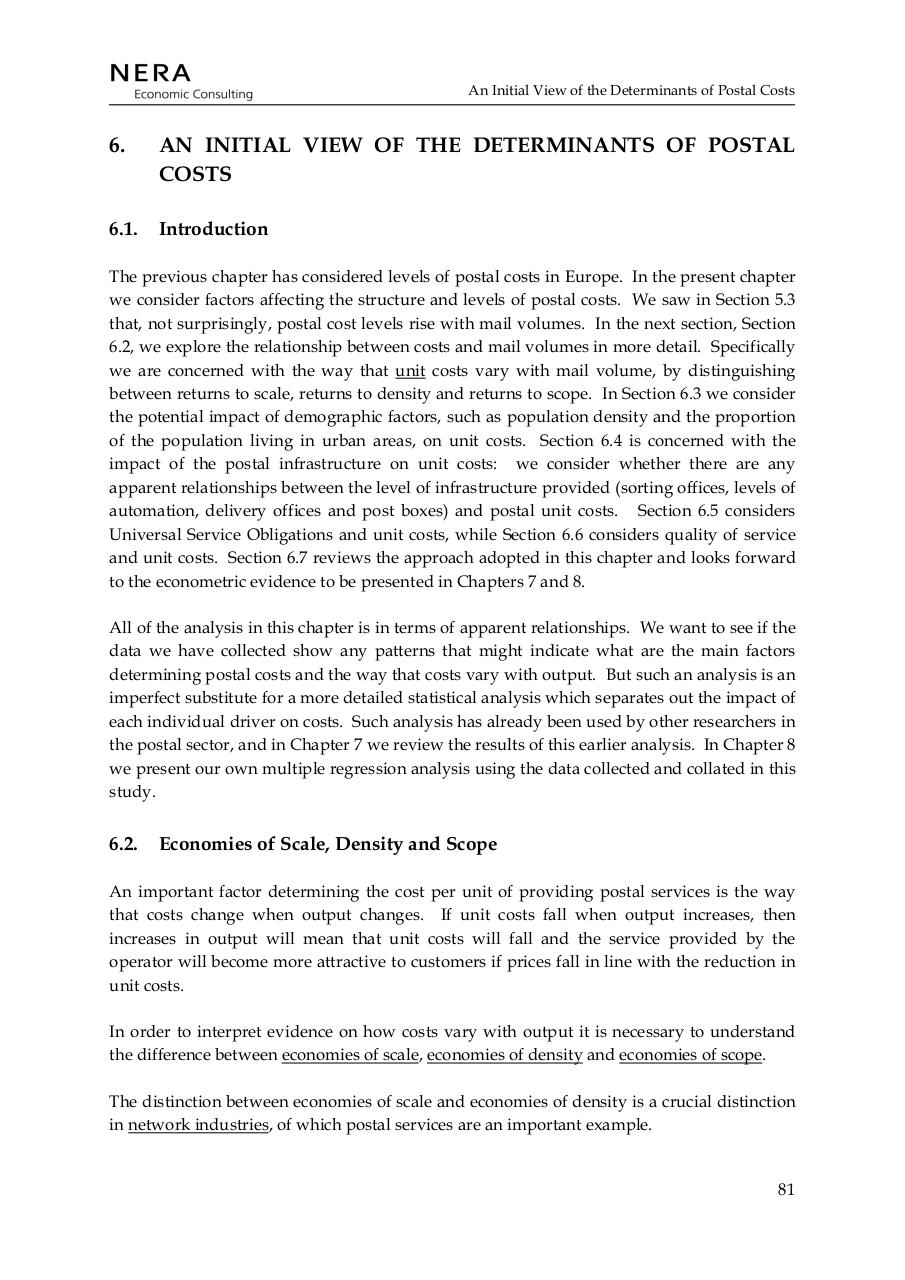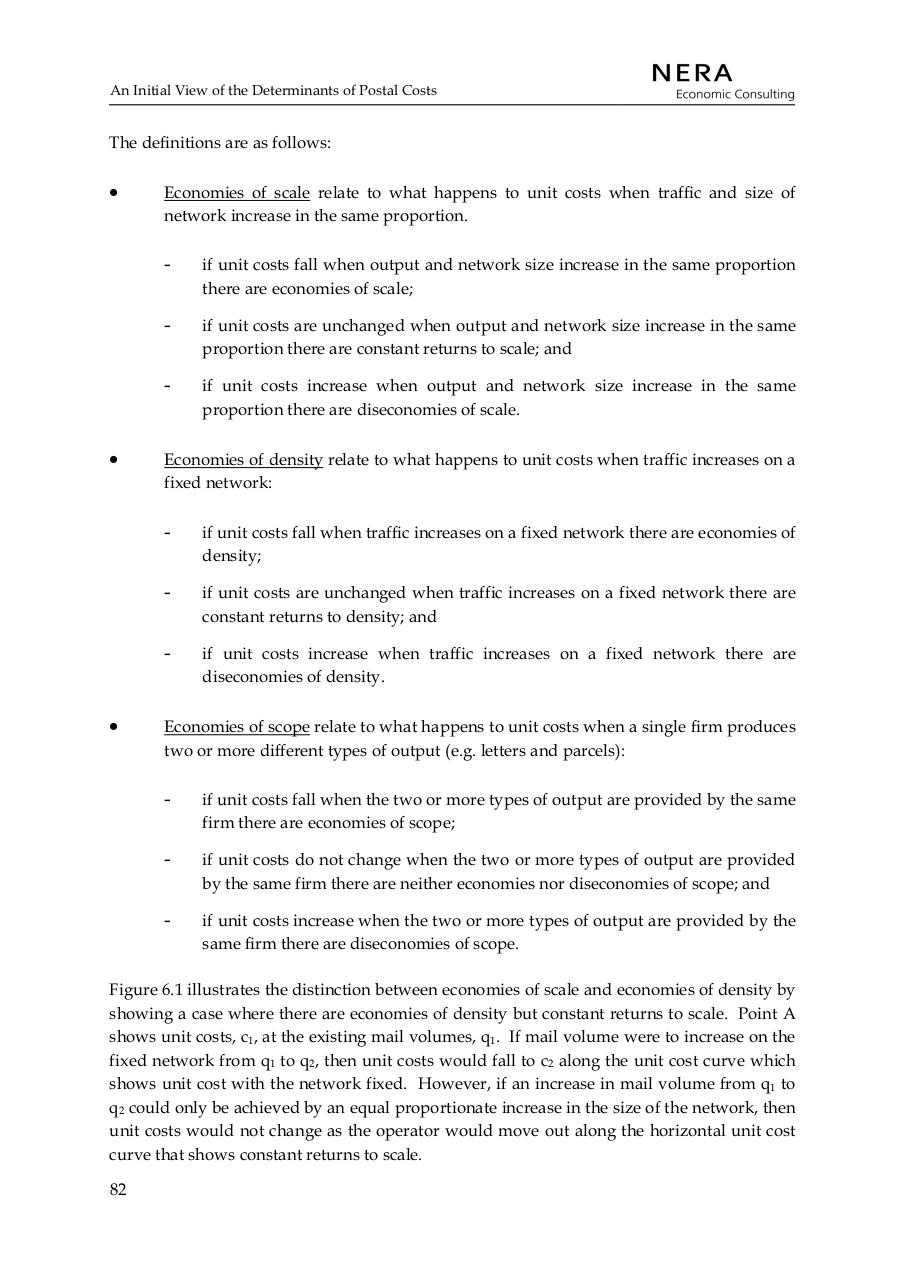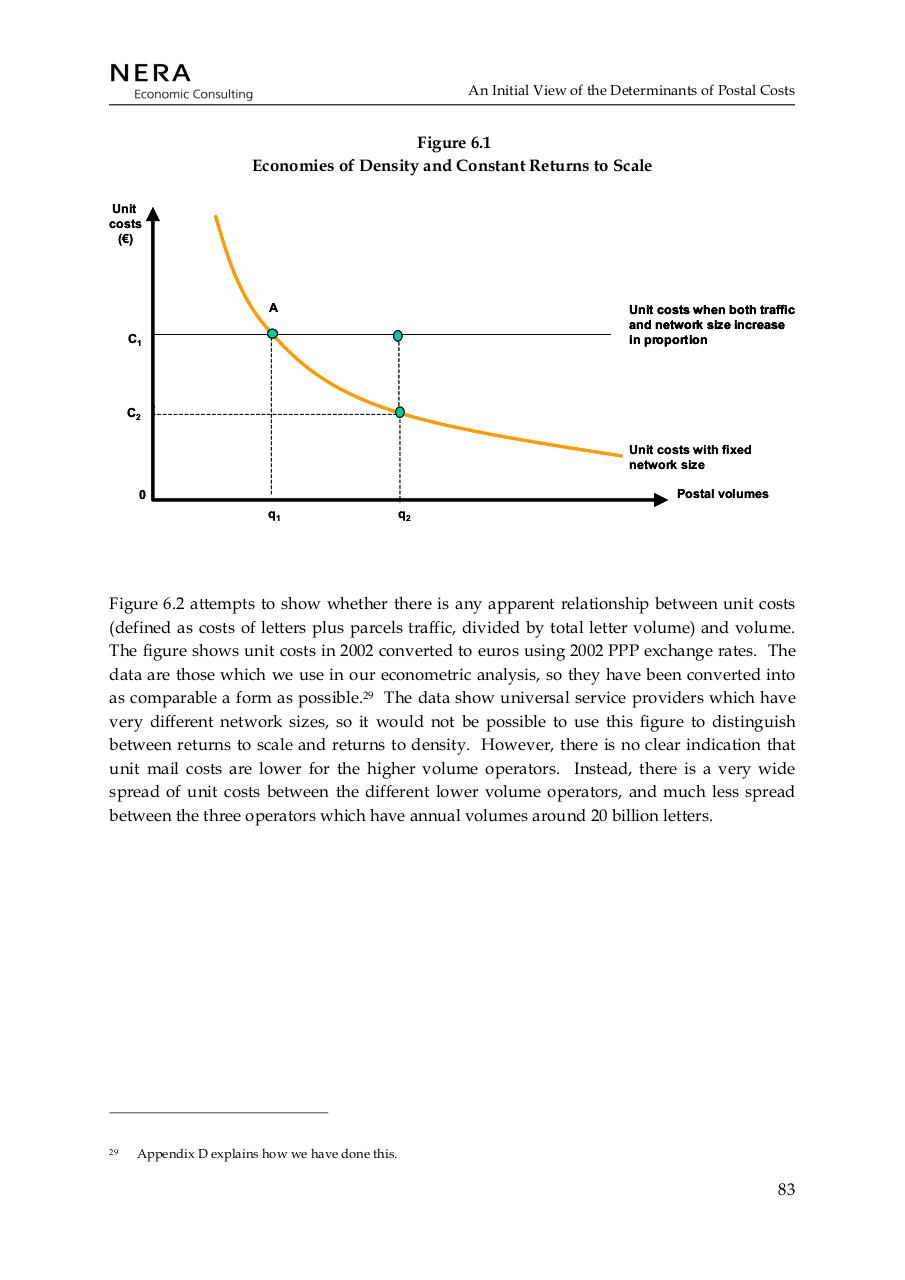# the economics of postal services NERA 07 2004 .pdf

### File information

Original filename: the economics of postal services - NERA- 07-2004.pdf

This PDF 1.7 document has been generated by PDFium, and has been sent on pdf-archive.com on 31/12/2015 at 21:31, from IP address 24.22.x.x. The current document download page has been viewed 327 times.
File size: 123 KB (3 pages).
Privacy: public file

the economics of postal services - NERA- 07-2004.pdf (PDF, 123 KB)

### Document preview

An Initial View of the Determinants of Postal Costs

6.

AN INITIAL VIEW OF THE DETERMINANTS OF POSTAL
COSTS

6.1.

Introduction

The previous chapter has considered levels of postal costs in Europe. In the present chapter
we consider factors affecting the structure and levels of postal costs. We saw in Section 5.3
that, not surprisingly, postal cost levels rise with mail volumes. In the next section, Section
6.2, we explore the relationship between costs and mail volumes in more detail. Specifically
we are concerned with the way that unit costs vary with mail volume, by distinguishing
between returns to scale, returns to density and returns to scope. In Section 6.3 we consider
the potential impact of demographic factors, such as population density and the proportion
of the population living in urban areas, on unit costs. Section 6.4 is concerned with the
impact of the postal infrastructure on unit costs: we consider whether there are any
apparent relationships between the level of infrastructure provided (sorting offices, levels of
automation, delivery offices and post boxes) and postal unit costs. Section 6.5 considers
Universal Service Obligations and unit costs, while Section 6.6 considers quality of service
and unit costs. Section 6.7 reviews the approach adopted in this chapter and looks forward
to the econometric evidence to be presented in Chapters 7 and 8.
All of the analysis in this chapter is in terms of apparent relationships. We want to see if the
data we have collected show any patterns that might indicate what are the main factors
determining postal costs and the way that costs vary with output. But such an analysis is an
imperfect substitute for a more detailed statistical analysis which separates out the impact of
each individual driver on costs. Such analysis has already been used by other researchers in
the postal sector, and in Chapter 7 we review the results of this earlier analysis. In Chapter 8
we present our own multiple regression analysis using the data collected and collated in this
study.

6.2.

Economies of Scale, Density and Scope

An important factor determining the cost per unit of providing postal services is the way
that costs change when output changes. If unit costs fall when output increases, then
increases in output will mean that unit costs will fall and the service provided by the
operator will become more attractive to customers if prices fall in line with the reduction in
unit costs.
In order to interpret evidence on how costs vary with output it is necessary to understand
the difference between economies of scale, economies of density and economies of scope.
The distinction between economies of scale and economies of density is a crucial distinction
in network industries, of which postal services are an important example.

81

An Initial View of the Determinants of Postal Costs

The definitions are as follows:

Economies of scale relate to what happens to unit costs when traffic and size of
network increase in the same proportion.

-

if unit costs fall when output and network size increase in the same proportion
there are economies of scale;

-

if unit costs are unchanged when output and network size increase in the same
proportion there are constant returns to scale; and

-

if unit costs increase when output and network size increase in the same
proportion there are diseconomies of scale.

Economies of density relate to what happens to unit costs when traffic increases on a
fixed network:

-

if unit costs fall when traffic increases on a fixed network there are economies of
density;

-

if unit costs are unchanged when traffic increases on a fixed network there are
constant returns to density; and

-

if unit costs increase when traffic increases on a fixed network there are
diseconomies of density.

Economies of scope relate to what happens to unit costs when a single firm produces
two or more different types of output (e.g. letters and parcels):

-

if unit costs fall when the two or more types of output are provided by the same
firm there are economies of scope;

-

if unit costs do not change when the two or more types of output are provided
by the same firm there are neither economies nor diseconomies of scope; and

-

if unit costs increase when the two or more types of output are provided by the
same firm there are diseconomies of scope.

Figure 6.1 illustrates the distinction between economies of scale and economies of density by
showing a case where there are economies of density but constant returns to scale. Point A
shows unit costs, c1, at the existing mail volumes, q1. If mail volume were to increase on the
fixed network from q1 to q2, then unit costs would fall to c2 along the unit cost curve which
shows unit cost with the network fixed. However, if an increase in mail volume from q1 to
q2 could only be achieved by an equal proportionate increase in the size of the network, then
unit costs would not change as the operator would move out along the horizontal unit cost
curve that shows constant returns to scale.
82

An Initial View of the Determinants of Postal Costs

Figure 6.1
Economies of Density and Constant Returns to Scale
Unit
costs
(€)

A

Unit costs when both traffic
and network size increase
in proportion

C1

C2
Unit costs with fixed
network size
Postal volumes

0
q1

q2

Figure 6.2 attempts to show whether there is any apparent relationship between unit costs
(defined as costs of letters plus parcels traffic, divided by total letter volume) and volume.
The figure shows unit costs in 2002 converted to euros using 2002 PPP exchange rates. The
data are those which we use in our econometric analysis, so they have been converted into
as comparable a form as possible.29 The data show universal service providers which have
very different network sizes, so it would not be possible to use this figure to distinguish
between returns to scale and returns to density. However, there is no clear indication that
unit mail costs are lower for the higher volume operators. Instead, there is a very wide
spread of unit costs between the different lower volume operators, and much less spread
between the three operators which have annual volumes around 20 billion letters.

29

Appendix D explains how we have done this.

83#### HTML Code

Copy the following HTML code to share your document on a Website or Blog

#### QR Code### Related keywords